Fall 2004

# Mathematics Math21b Fall 2004

## Linear Algebra and Differential Equations

Office: SciCtr 434
Email: knill@math.harvard.edu# Imagefilters and Linear Algebra

Note (added 2008): if you use mathematica 6, replace "Display" by "Show".A color image can be described by three matrixes R,G,B, where Rij, Gij and Bij are the amount of red, green and blue in the picture at position (i,j). It is custom to encode with an integer from 0 to 255, which can also be seen as 1 byte = 8 bits because 28=256. The picture to the left with width 2 and height 2 can be described by three 2x2 matrices: ``` | 0 255 | | 0 0 | | 255 0 | R = | | G = | | B = | | | 0 255 | | 255 255 | | 0 0 | ``` The data can also be described by one matrix alone, where we give the (rgb) value at each point ``` | [0 0 1] [1 0 0] | | blue red | A = | | = | | | [0 1 0] [1 1 0] | | green yellow | ```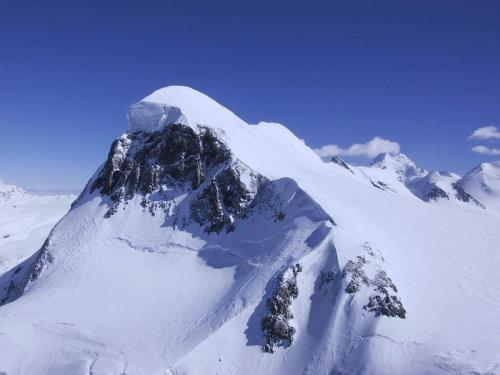Evenso the range of the coordinates is limited and discretized, we can think of a picture as an element in a linear space. We can scale pictures, project onto subspaces or make linear combinations. To illustrate this, we use Mathematica, which allows very easily to import and export pictures. The pictures are matrices which can be manipulated. For example ```A=Import["zermatt.jpg"]; ``` imports a picture of a mountain near Zermatt in Switzerland.
 Here is a Mathematica procedure, which takes such imported image data and applies a filter ```ApplyFilter[F_,A_]:=Graphics[Raster[F[A[[1,1]]],A[[1,2]],A[[1,3]],A[[1,4]]],A[],A[],A[]]; ``` Mathematica keeps more than just the color matrix. Important to know is that A[[1,1]] is the matrix which contains the RGB values of each picture. For example ```A = Graphics[Raster[{{{0, 255, 0}, {255, 255, 0}}, {{0, 97, 255}, {255, 0, 0}}}, {{0, 0}, {2, 2}}, {0, 255}, ColorFunction -> RGBColor], ImageSize -> {2, 2}, PlotRange -> {{0, 1}, {1, 2}}, AspectRatio -> Automatic] ``` is, what Mathematica has stored about the 2x2 pixel picture above. We access the RGB matrix with A[[1,1]].

### Scaling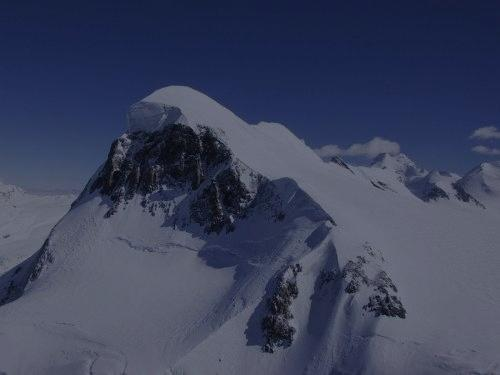Here is an example, which "scales" a picture: ```A=Import["zermatt.jpg"]; Dim[x_]:=Round[x/2]; B1=ApplyFilter[Dim,A]; Brighten[x_]:=Round[120+2x/3]; B2=ApplyFilter[Brighten,A]; Display["dimmed.jpg",B1,"JPEG"]; Display["brightend.jpg",B2,"JPEG"]; ```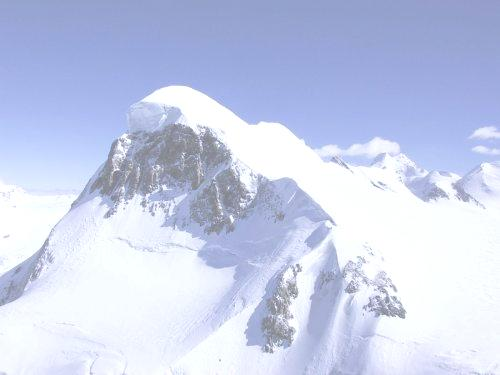### Projection

Here is an example of a projection onto the red green or blue planes:
```red[{r_,g_,b_}]:={r,0,0}; Red[S_]:=Table[red[S[[i,j]]],{i,Length[S]},{j,Length[S[]]}];
green[{r_,g_,b_}]:={0,g,0}; Green[S_]:=Table[green[S[[i,j]]],{i,Length[S]},{j,Length[S[]]}];
blue[{r_,g_,b_}]:={0,0,b}; Blue[S_]:=Table[blue[S[[i,j]]],{i,Length[S]},{j,Length[S[]]}];

B1=ApplyFilter[Red,A]; B2=ApplyFilter[Green,A]; B3=ApplyFilter[Blue,A];
Display["red.jpg",B1,"JPEG"]; Display["green.jpg",B2,"JPEG"]; Display["blue.jpg",B3,"JPEG"];
```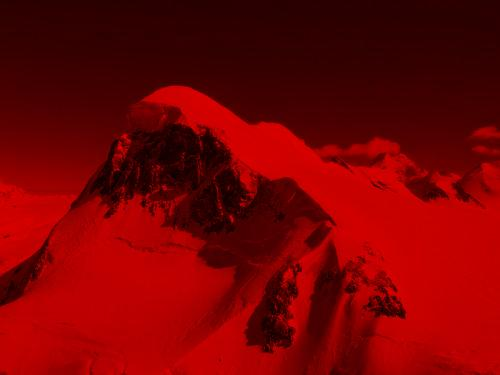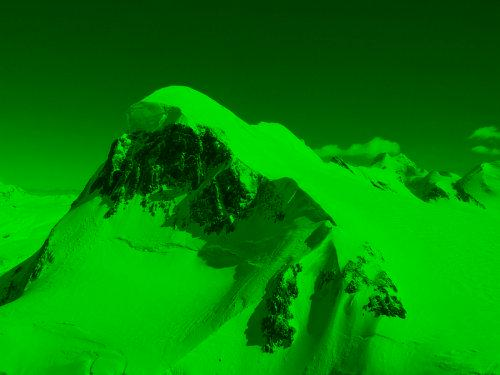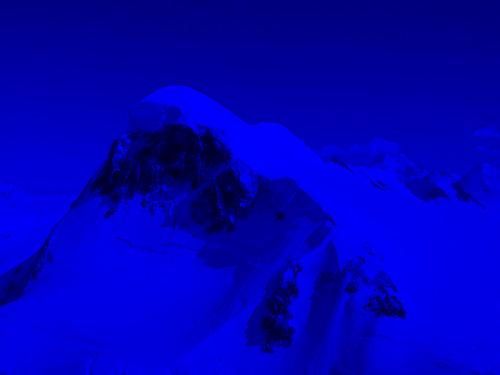### Rotation and Reflection

Here is an example of a rotation or reflection in color space.
```rotate[{r_,g_,b_}]:={b,r,g}; Rotate[S_]:=Table[rotate[S[[i,j]]],{i,Length[S]},{j,Length[S[]]}];
reflect[{r_,g_,b_}]:={255-r,255-g,255-b}; Reflect[S_]:=Table[reflect[S[[i,j]]],{i,Length[S]},{j,Length[S[]]}];
B4=ApplyFilter[Rotate,A];
B5=ApplyFilter[Reflect,A];
Display["rotate.jpg",B4,"JPEG"];
Display["reflect.jpg",B5,"JPEG"];
```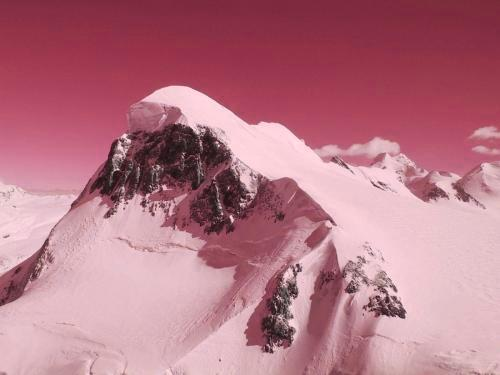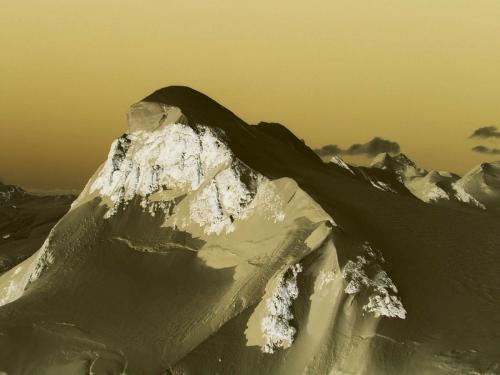### Linear Combinations

The procedure
```Combine[F_,U_,V_]:=Graphics[Raster[F[U[[1,1]],V[[1,1]]],U[[1,2]],U[[1,3]],U[[1,4]]],U[],U[],U[]];
```
allows to combine two pictures using a function f[x,y].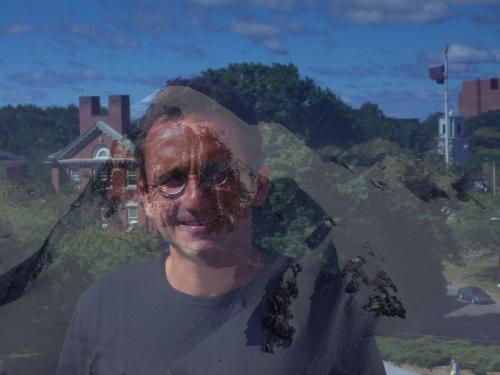Here is an example on how to take two pictures and make a simple linear combination, where we take 1/3 of a mountain and 2/3 of a person and mix it up.
```A1=Import["zermatt.jpg"]; A2=Import["oliver.jpg"];
LinearCombination[a_,b_]:=Function[{x,y},a*x+b*y];
B=Combine[LinearCombination[1/3,2/3],A1,A2];
Display["mixture.jpg",B,"JPEG"];
```
 We can do that for several linear combinations and animate that.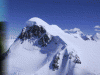Note that each picture is in a huge dimensional space. The pictures we dealt with here had size 500x375 which are 187'500 pixels. Each pixels is encoded by 3 bytes so that this picture weights 1/2 megabytes. Indeed, uncompressed, the pictures needs this amount of space to be stored. Here is an other example of a linear combination of two vectors rsp. pictures. The combination amoung depends now on the pixel position.```F1[a_,b_]:=Function[{x,y}, Module[{}, m=Length[x[]]; (* width *) n=Length[x]; (* height *) z=x; Do[ If[jj>a*m, K=(j-a*m)/(b*m-a*m)]; If[j>b*m, K=1]; z[[i,j]]=Round[K*x[[i,j]]+(1-K)*y[[i,j]]], {i,n},{j,m} ]; z ] ] B=Combine[F1[1/3,2/3],A1,A2]; Display["leftrighttransition.jpg",B,"JPEG"]; ```

### An other filter```F2[r1_,r2_]:=Function[{x,y}, Module[{}, m=Length[x[]]; mc=m/2; n=Length[x]; nc=n/2; rr1=(Min[m,n]*r1)^2; rr2=(Min[m,n]*r2)^2; z=x; Do[ rr=(i-nc)^2+(j-mc)^2; K=0; If[rr1<=rr<=rr2, K=(rr-rr1)/(rr2-rr1)]; If[rr>rr2, K=1]; z[[i,j]]=Round[K*x[[i,j]]+(1-K)*y[[i,j]]], {i,n},{j,m} ]; z ] ] B=Combine[F2[1/10,1/2],A1,A2]; Show["circle.jpg",B,"JPEG"]; ```

To try it out, donwload the two pictures as well as the code picturemerge.m and run it. You don't want to run Mathematica in the kiddie mode (graphical user interface) don't you?.
```math < picturemerge.m >out  &
```
You have to wait until the newly produced files are in the directory. There are many advantages not to use the graphical Mathematica interface
 you can not use your favorate editor a GUI interface is less stable and uses valuable resources from the commandline, you can run the above code for huge pictures, leave it processing in the background, run it on different server.

To run Mathematica from the commandline in OSX, add something like
```alias math='/Applications/Mathematica\ 5.0.app/Contents/MacOS/MathKernel'
```